Courses

# Test: System Of Particles And Rotational Motion 1 - From Past 28 Years Questions

## 15 Questions MCQ Test Physics 28 Years Past year papers for NEET/AIPMT Class 11 | Test: System Of Particles And Rotational Motion 1 - From Past 28 Years Questions

Description
This mock test of Test: System Of Particles And Rotational Motion 1 - From Past 28 Years Questions for NEET helps you for every NEET entrance exam. This contains 15 Multiple Choice Questions for NEET Test: System Of Particles And Rotational Motion 1 - From Past 28 Years Questions (mcq) to study with solutions a complete question bank. The solved questions answers in this Test: System Of Particles And Rotational Motion 1 - From Past 28 Years Questions quiz give you a good mix of easy questions and tough questions. NEET students definitely take this Test: System Of Particles And Rotational Motion 1 - From Past 28 Years Questions exercise for a better result in the exam. You can find other Test: System Of Particles And Rotational Motion 1 - From Past 28 Years Questions extra questions, long questions & short questions for NEET on EduRev as well by searching above.
QUESTION: 1

### A constant torque of 1000 N-m turns a wheel of moment of inertia 200 kg-m2 about an axis through its centre. Its angular velocity after 3 seconds is 

Solution:

τ = 1000N - m, I = 200 Kg-m2
∴ Iα = 1000
⇒ α = 1000 / 200 = 5 rad/sec2
ω = ωo + αt = 0 + 3 x 5 = 15 rad/s

QUESTION: 2

### A wheel of radius 1m r olls for war d half a revolution on a horizontal ground. The magnitude of the displacement of the point of the wheel initially in contact with the ground is 

Solution: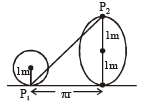Linear distance moved by wheel in half revolution = πr. Point P1 after half revolution reaches at P2 vertically 2m above the ground.

∴   Displacement P1P2
= √π2r2 + 22 = √π2 + 4     [∵ r = 1m]

QUESTION: 3

### If the linear density (mass per unit length) of a rod of length 3m is proportional to x, where x is the distance from one end of the rod, the distance of the centre of gravity of the rod from this end is

Solution:

Consider an element of length dx at a distance x from end A.
Here,  mass per unit length λ of rod
λ ∞ x ⇒ λ = kx
∴ dm = λdx = kx dx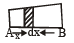Position of centre of gravity of rod from end A.
L          L
xCG = ∫ xdm / ∫ dm
0          0
3                3
∴ xCG = ∫ x(kx dx) / ∫ kx dx = [x3 / 3]03 / [x2 / 2]03
0                0
= (3)3 / 3 / (3)3 / 2 = 2m

QUESTION: 4

A disc is rotating with angular velocity ω. If a child sits on it, what is conserved ? 

Solution:

If external torque is zero, angular momentum remains conserved. [External torque is zero because the weight of child acts downward]
L = Iω = constant

QUESTION: 5

A composite disc is to be made using equal masses of aluminium and iron so that it has as high a moment of inertia as possible. This is possible when 

Solution:

Density of iron > density of aluminium moment of inertia = ∫ r2 dm.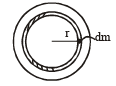∴ Since, ρiron > ρaluminium

So, whole of aluminium is kept in the core and the iron at the outer rim of the disc.

QUESTION: 6

When a mass is rotating in a plane about a fixed point, its angular momentum is directed along : 

Solution: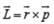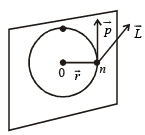By right hand screw, rule, the direction of L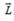is ⊥ to the plane containing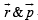.
The mass is rotating in the plane, about a fixed point, thus this plane will containand the direction of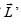will be ⊥ to the this plane.

QUESTION: 7

Two persons of masses 55 kg and 65 kg respectively, are at the opposite ends of a boat.The length of the boat is 3.0 m and weighs 100 kg. The 55 kg man walks up to the 65 kg man and sits with him. If the boat is in still water the centre of mass of the system shifts by : 

Solution: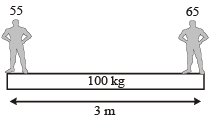There is no external force so centre of mass of the system will not shift

QUESTION: 8

ABC is an equilateral triangle with O as its centre.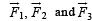represent three forces acting along the sides AB, BC and AC respectively. If the total torque about O is zero the magnitude of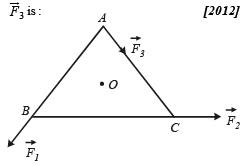Solution:

F1 x + F2 x = F3 x
F3 = F1 + F2

QUESTION: 9

A circular platform is mounted on a frictionless vertical axle. Its radius R = 2 m and its moment of inertia about the axle is 200 kgm2. It is initially at rest. A 50 kg man stands on the edge of the platform and begins to walk along the edge at the speed of 1 ms–1 relative to the ground. Time taken by the man to complete one revolution is :

Solution:

By applying the law of conservation of angular momentum,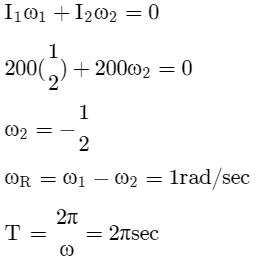QUESTION: 10

The moment of inertia of a uniform circular disc is maximum about an axis perpendicular to the disc and passing through : [2012M]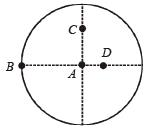Solution:

According to parallel axis theorem of the moment of Inertia    I = Icm + md2

d is maximum for point B so Imax about B.

QUESTION: 11

Three masses are placed on the x-axis : 300g at origin, 500g at x = 40cm and 400g at x = 70 cm.The distance of the centre of mass from the origin is : [2012M]

Solution:

Xcm = m1x1 + m2x2 + m3x3 / m1 + m2 + m3
Xcm = 300 x (0) + 500(40) + 400 x 70 / 300 + 500 + 400
Xcm = 500 x 40 + 400 x 70 / 1200
Xcm = 50 + 70 / 3 = 120 / 3 = 40 cm

QUESTION: 12

A small object of uniform density rolls up a curved surface with an initial velocity  ‘ν’. It reaches upto a maximum height of 3v2 / 4g with respect to the initial position. The object is a  [NEET 2013]

Solution: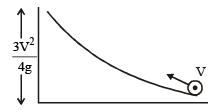From law of conservation of mechanical energy
1 / 2 Iω2 + 0 + 1 / 2 mv2 = mg x 3v2 / 4g
⇒ 1 / 2 Iω2 = 3 / 4 mv2 - 1 / 2 mv2
= mv2 / 2(3 / 2 - 1)
or, 1 / 2 I V2 / R2 = mv2 / 4 or, I = 1 / 2 mR2
Hence, object is a disc.

QUESTION: 13

A rod PQ of mass M and length L is hinged at end P. The rod is kept horizontal by a massless string tied to point Q as shown in figure. When string is cut, the initial angular acceleration of the rod is [NEET 2013]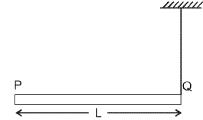Solution: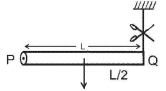Weight of the rod will produce the torque
τ = mg x L / 2 = I α = mL2 / 3 α
[∵ Irod = ML2 / 3]

Hence, angular acceleration α = 3g / 2L

QUESTION: 14

Two discs are rotating about their axes, normal to the discs and passing through the centres of the discs. Disc D1 has 2 kg mass and 0.2 m radius and initial angular velocity of  50 rad s–1. Disc D2 has 4kg mass, 0.1 m radius and initial angular velocity of 200 rad s–1. The two discs are brought in contact face to face, with their axes of rotation coincident. The final angular velocity (in rad s–1) of the system is [NEET Kar. 2013]

Solution:

Given: m1 = 2 kg m2 = 4 kg

r1 = 0.2 m r2 = 0.1 m

As,   I1W1 = I2W2 = Constant
∴ Wf = I1W1 + I2W2 / I1 + I2 = 1 / 2 m1r1w+ 1 / 2 m2r2w2 / 1 / 2 m1r12 + 1 / 2 m2r22

By putting the value of m1, m2, r1, r2 and solving we get = 100 rad s–1

QUESTION: 15

The ratio of radii of gyration of a circular ring and a circular disc, of the same mass and radius, about an axis passing through their centres and perpendicular to their planes are [NEET Kar. 2013]

Solution:

∵ I = MK2 ∴ K = √I / M
Iring = MR2 and Idisc = 1 / 2 MR2
K1 / K2 = √I1 / I2 = √MR2 / (MR2 / 2) = √2 : 1